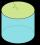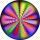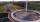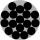# Circle - easy 2

The circle has a radius 6 cm. Calculate:

d =  3 cm
p =  37.7 cm
T =  113.1 cm2

### Step-by-step explanation:Did you find an error or inaccuracy? Feel free to write us. Thank you!#### You need to know the following knowledge to solve this word math problem:

We encourage you to watch this tutorial video on this math problem:

## Related math problems and questions:

• Curved surface area CSAA cylinder 5cm high has a base radius(7/2) cm. Calculate the curved surface area.
• 22/7 circleCalculate approximately the area of a circle with radius 20 cm. When calculating π, use 22/7.Find the radius of the circle with area S = 200 cm².
• Circle areaCalculate the circle area with a radius of 1.2 m.
• AnnulusThe radius of the larger circle is 8cm, the radius of smaller is 5cm. Calculate the contents of the annulus.
• ShapePlane shape has a maximum area 677 mm2. Calculate its perimeter if perimeter is the smallest possible.Calculate the radius of the circle whose length is 107 cm larger than its diameterCalculate the radius of the quadrant, which area is equal to area of circle with radius r = 15 cm.
• ParallelogramCalculate the area and perimeter of a parallelogram whose two sides are long a=24 cm b=22 cm and height ha = 6 cm long.
• CircleHow big is an area of a circle if its circumference is 51.2 cm?
• Area and perimeter 2Find the area and the perimeter of a rectangle of length 45 1/2 cm and breadth 16 2/3 cm.
• AnnulusCalculate the area of two circles annulus k1 (S, 3 cm) and k2 (S, 5 cm).
• Four circles1) Calculate the circle radius if its area is 400 cm square 2) Calculate the radius of the circle whose circumference is 400 cm. 3) Calculate circle circumference if its area is 400 cm square 4) Calculate the circle's area if perimeter 400 cm.
• Perimeter to areaCalculate the area of a circle with a perimeter of 15 meters.
• Perimeter of squareThe square has a circumference 17cm. What is its area?
• CalculateCalculate the area of a circle whose radius is 5 dm.
• Diameter to areaFind the area of a circle whose diameter is 26cm.